## Output Devices

Group assignment

### Group assignment:

measure the power consumption of an output device

Power: It is the amount of energy transferred or converted per unit time. In the International System of Units, the unit of power is the watt, equal to one joule per second. In older works, power is sometimes called activity. Power is a scalar quantity.

The instantaneous electrical power P delivered to a component is given by P(t)=V(t)xI(t) Where

P(t)= is the instantaneous power, measured in watts (joules per second)
V(t)= is the potential difference (or voltage drop) across the component, measured in volts
I(t)= is the current through it, measured in amperes

Voltage: Voltage, electric potential difference, electric pressure or electric tension is the difference in electric potential between two points. The difference in electric potential between two points (i.e., voltage) in a static electric field is defined as the work needed per unit of charge to move a test charge between the two points. In the International System of Units, the derived unit for voltage is named volt.
In SI units, work per unit charge is expressed as joules per coulomb, where 1 volt = 1 joule (of work) per 1 coulomb (of charge). The official SI definition for volt uses power and current, where 1 volt = 1 watt (of power) per 1 ampere (of current).

Current: An electric current is the rate of flow of electric charge past a point or region. An electric current is said to exist when there is a net flow of electric charge through a region.In electric circuits this charge is often carried by electrons moving through a wire. It can also be carried by ions in an electrolyte, or by both ions and electrons such as in an ionized gas (plasma).
The SI unit of electric current is the ampere, which is the flow of electric charge across a surface at the rate of one coulomb per second. The ampere (symbol: A) is an SI base unit. Electric current is measured using a device called an ammeter

Here we have used the Hxahust fan and 12 volt (DC) submersible water pump. so for the group assignment I tried to calculate the power of these two devices. Input refers to the amount of energy put into a device, and outputrefers to the amount of energy that comes out. A device may change the type of energy but not the amount. For example, a light bulb’s input energy is the form of electrical energy, and its output energy is in the form of light and heat.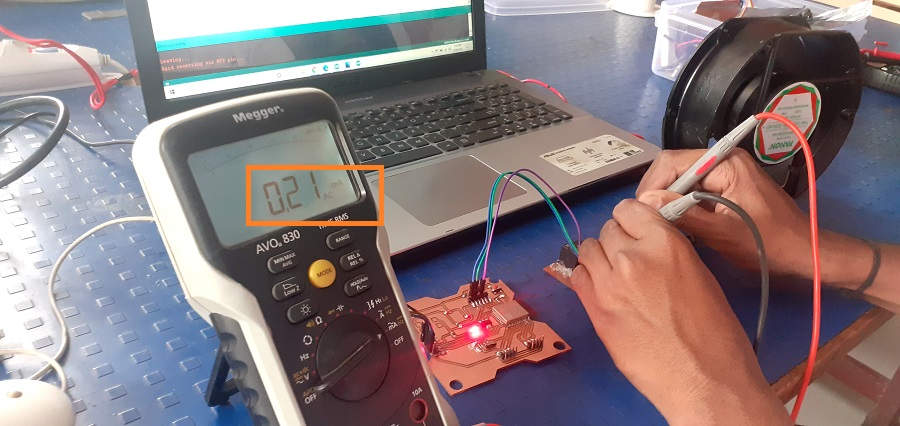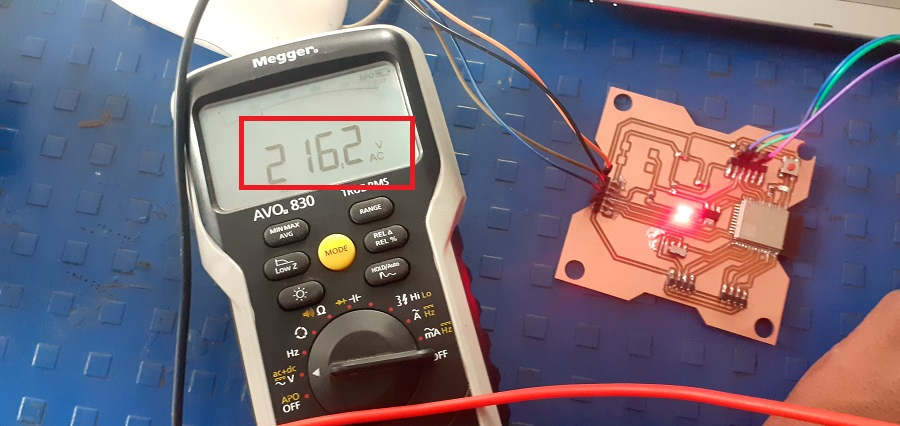Calculating Power for AC Exahust Fan

I have used the multimeter for measureing the AC current and AC voltage required for the exahust fan as shown in images

Measuring AC Current

Measuring AC voltage
Current(I)=0.21mA, Voltage(V)=216.2 Volt

Power(P)=0.21 x10x216.2

Power(P)=0.045 W

Calculating Power for 12VDC submersible water pump.

The power of water can be calculate in two ways in presence of air and in presence of water.

Current(I)=0.146 A, Voltage(V)=12 Volt

Power(P)=0.146x12

Power(P)=1.752 W

`In presence of air`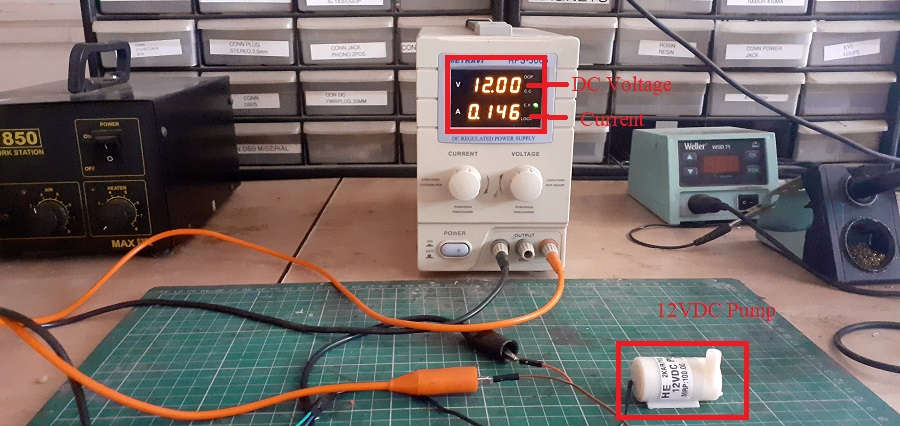`In presence of air`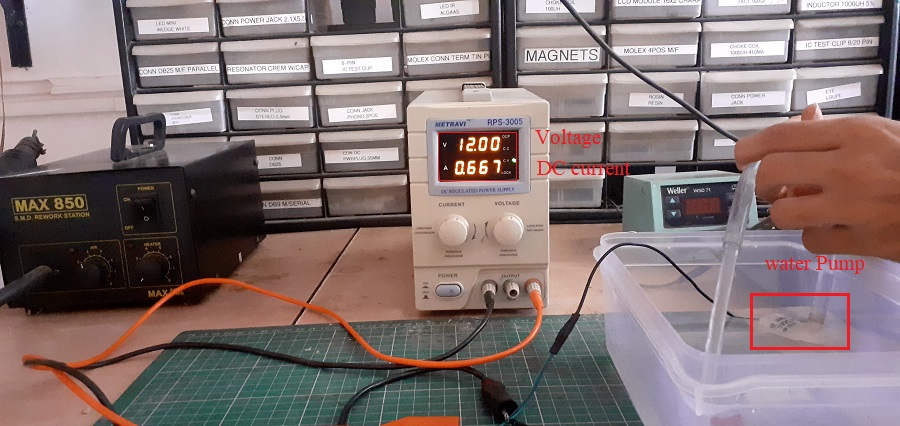Current(I)=0.667 A, Voltage(V)=12 Volt

Power(P)=0.667x12

Power(P)=8.004 W

Power Consumption Calculations for 16*2 LCD Display:

On ATMega-328P Board:
I have tested the Power Consumption for 16*2 LCD on ATMEGA 328P where 5V supply was given to the Board. I connected the Multimeter in Series with the Vcc. I connected the GND of LCD to GND of the ATMEGA-328P board. I connected the Vcc of the Board to one of the Terminals of the Multimeter and the other Pin of Multimeter to the Vcc of the LCD. This how the Multimeter was connected in Series with the LCD and ATMega Board. I connected the Black Pin of the Multimeter to the Common and Red Pin to the 100mA. I also rotated the knob on the mA/Hz to check the Current.

The reading on the Multimeter was 20.85 mA. I have given a Voltage of 5V. So, the Power consumption will be P= 5 x 20.85 x 10^-3 = 0.1 Watt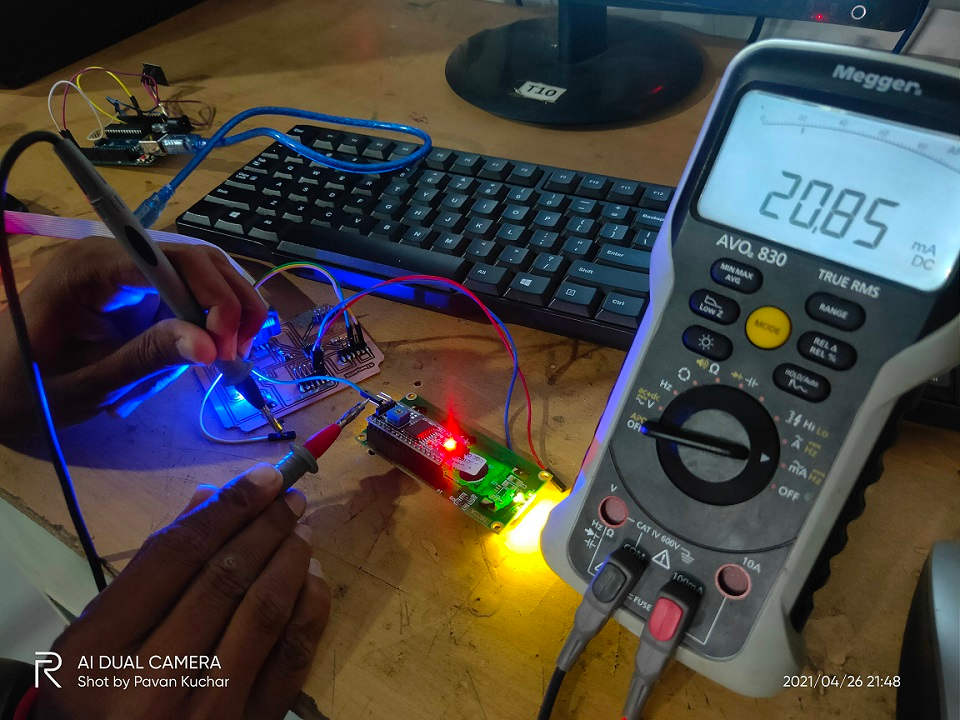Current (mA) Readings of LCD at 5 Volt Supply

On my ESP-32 Board:
I made the connections in Series like done for ATMEGA earlier. Only thing is I have provided the 3.3 Volts supply.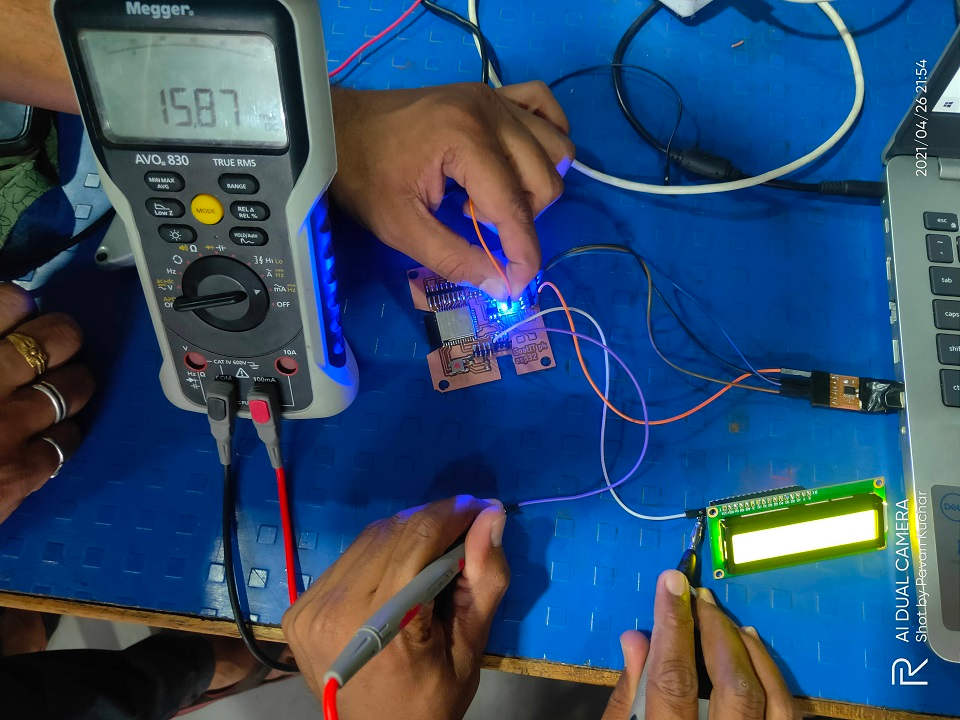Current (mA) Readings of LCD at 3.3 Volt Supply

The reading on the Multimeter was 15.87 mA. I have given a Voltage of 3.3V. So, the Power consumption will be P= 3.3 x 15.87 x 10^-3 = 0.052371 Watt

I thought that the Power Consumption of LCD would remain the same. But, it has different Current readings for 3.3V and 5V supply and thus the Power Consumption differs. This might be because of the Contrast of the BackLight of the LCD as it changes on different voltage.

Calculations of Power Consumption for NIMA-17 Stepper Motor:-

I have measure the Required power Consumption for Stepper motor Here.when motor is Controlling through Atmega328p Board with A4988 motor Driver.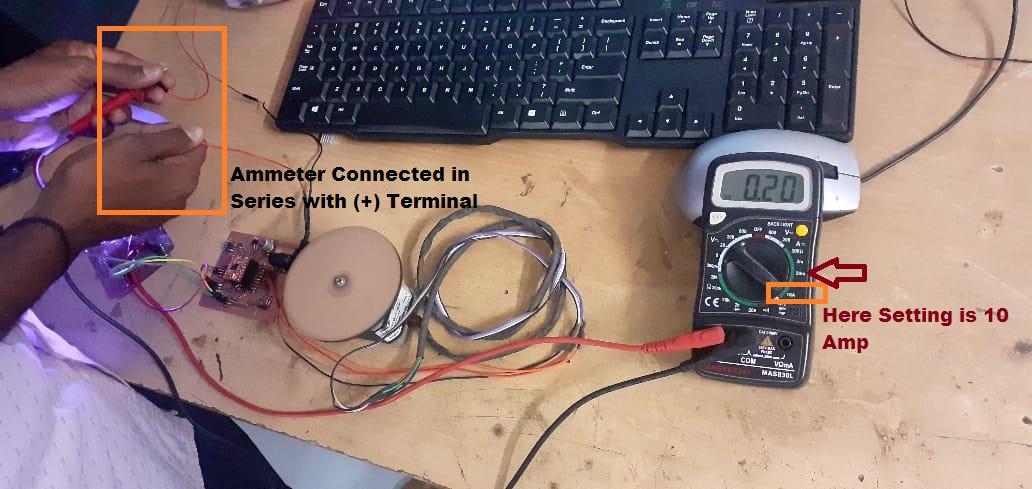Measuring the Current Required for Stepper Motor

For controllling the stepper motor through any microcontroller board we required driver circuit and this driver circuit required 12Volts Dc power from external Source.
Then I have measure the required current for Biopolar stepper motor by connecting Ammmeter in series with the possitive supply terminal of external voltage source i.e. 12 Volts. Beacuse for calculating the power consumption of given Motor i required the values of Voltage and Current.

The total current flowing through the circuit is 0.20 Amp When motor is on noload conditions for stepper motor is

So, here it is observed that the total power comsume by the stepper motor as per formulas i.e. p(t)=v(t)*i(t)
P(t)= 12*0.20=2.4 Watt